### Home > CAAC > Chapter 2 > Lesson 2.2.1 > Problem2-96

2-96.
1. Copy and simplify the following expressions by combining like terms. Homework Help ✎

1. y + 2x − 3+ 4x 2 + 3x − 5y

2. 2x − 6x 2 + 9 − 1 − x − 3x

3. 2y 2 + 30x − 5y 2 + 4x − 4y − y

4. −10 + 3xy − 3xy + y 2 +10 − y 2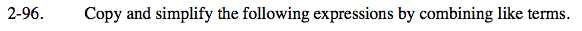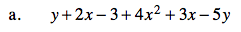Rearrange so that like terms are together, and so that terms are in order of power. Then, combine like terms.

4x² + 5x − 4y − 3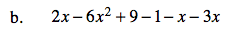Follow the steps in part (a).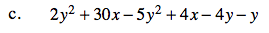Follow the steps in part (a).

3y² + 34x − 5y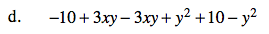Follow the steps in part (a).

Don't lose track of negative signs.